Total marks: --
Total time: --
INSTRUCTIONS
(1) Assume appropriate data and state your reasons
(2) Marks are given to the right of every question
(3) Draw neat diagrams wherever necessary

Answer any one question from Q1 and Q2
1 (a) Apply mesh analysis and determine the currents I1 and I2.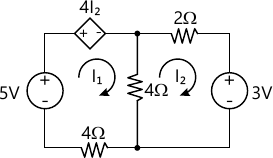6 M
1 (b) Explain the following terms with example:
i) Oriented graph.
ii) Tieset matrix.
iii) f-cutset matrix.
6 M

2 (a) Consider the circuit given below:
i) Obtain Thevenin's equivalent circuit.
ii) What load should be connected between terminals A-B for maximum power- transfer to the load?
iii) Calculate the maximum power transferred to the load.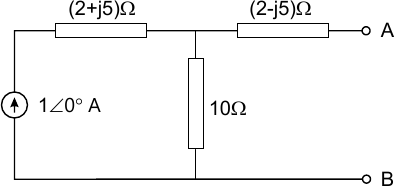6 M
2 (b) For the circuit and its graph shown below:
i) Write a tie-set schedule for the tree [4, 5, 6].
ii) Find the branch-impedance matrix.
iii) Obtain the loop impedance matrix.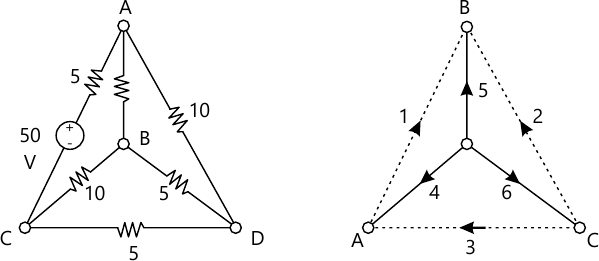6 M

Answer any one question from Q3 and Q4
3 (a) For the circuit shown below, find the voltage vc at t=200 μs. Find the expression for the current through capacitor ic(t).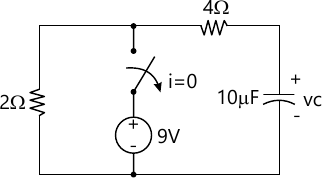6 M
3 (b) Define the term Quality factor. Prove for a series RLC resonant circuit $f_0 = \sqrt{f_1 f_2}$
6 M

4 (a) Derive the expression for the current i(t) for the series RL circuit shown below: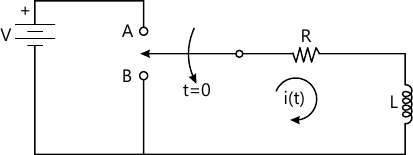6 M
4 (b) A series resonant circuit has a bandwidth of 100 Hz and contains a 20 mH inductance and a 20 μF capacitance. Determine:
i) f0
ii) Q0 and
iii) Impedance Z at resonance.
6 M

Answer any one question from Q5 and Q6
5 (a) For any symmetrical network, prove that the characteristics impedanceZ0 is the geometric-mean of open & short circuit impedances.
6 M
5 (b) Design a constant-k T-type low pass filter with following specifications: Design resistance R0 = 560 ? and cut-off frequency fc = 2KHz. Also determine the frequency at which the attenuation offered by the filter is 17.372dB.
7 M

6 (a) Design a symmetrical π attenuator with following specifications: Attenuation = 6dB and characteristic resistance of 6 dB. Draw a neat diagram of the properly terminated attenuator showing the component values.
6 M
i) State the limitations of prototype filters.
ii) Explain how these limitations are overcome using m-derived filters.
iii) Draw the block diagram of composite filters.
7 M

Answer any one question from Q7 and Q8
7 (a) Find z-parameters for the two-port network shown below. State whether the network is symmetrical/reciprocal.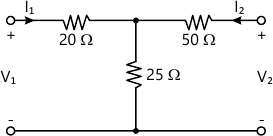6 M
7 (b) Consider the RC network shown below.
i) Draw the s-domain equivalent circuit.
ii) Find the transfer function $H(S)= \dfrac {V_{out}(S)}{V_i (S)}$ iii) Find the poles and zeros of the function H(s) and
iv) State whether the snystem is stable or not.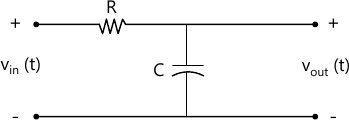7 M

8 (a) Find the four short circuit admittance parameters for the resistive two port network. Determine whether the network is symmetrical/reciprocal.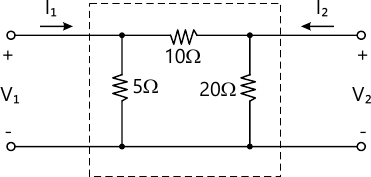6 M
8 (b) State and explain:
i) Driving point functions for one port networks.
ii) Driving point and transfer functions for two port networks.
7 M

More question papers from Network Theory